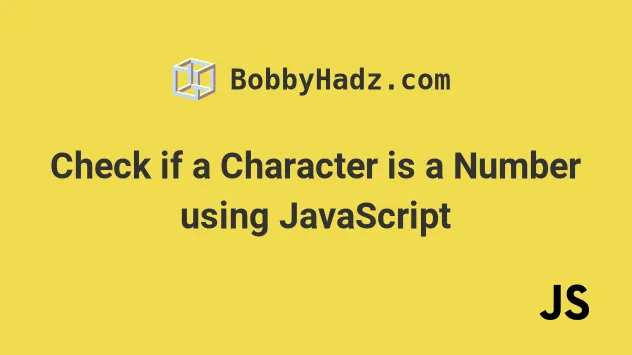# Check if a Character is a Number using JavaScriptFri Oct 22 20213 min readPhoto by Kalen Emsley

## Check if Character in String is a Number#

To check if a character is a number, pass the character as a parameter to the `isNaN()` function. The function checks if the provided value is `NaN` (not a number). If the function returns `false`, then the character is a valid number.

index.js
```Copied!```function isNumber(char) {
if (typeof char !== 'string') {
return false;
}

if (char.trim() === '') {
return false;
}

return !isNaN(char);
}

const str = 'a1';
console.log(isNumber(str)); // 👉️ false
console.log(isNumber(str)); // 👉️ true
console.log(isNumber('123')); // 👉️ true
console.log(isNumber('')); // 👉️ false
console.log(isNumber(undefined)); // false
``````

We first check if the value passed to the function is not of type `string`, in which case we return `false` straight away.

Then we check if the provided value is an empty string or contains only whitespace, in which case we also return `false`.

Finally, we use the isNaN function to check if the provided character is not a number.

We use the logical NOT (!) operator to negate the value returned from the `isNaN` (is not a number) function.

The `isNaN` function tries to convert the string into a number and if it fails, it returns `true`.

index.js
```Copied!```console.log(isNaN('a')); // 👉️ true
console.log(isNaN('')); // 👉️ false
console.log(isNaN('   ')); // 👉️ false
``````
This seems quite confusing at first, but the `isNaN` function converts an empty string or a string containing spaces to a number and gets a value of `0`, so it returns `false`.
index.js
```Copied!```console.log(Number('')); // 👉️ 0
console.log(Number('   ')); // 👉️ 0
``````

This is why we use the `trim()` method to trim the string and verify that it's not an empty string.

We know that if the `isNaN` function gets called with a string that contains at least 1 character and returns `true`, then the string is NOT a valid number.

Conversely, if the `isNaN` function gets called with a string that contains at least 1 character and returns `false`, then the string is a valid number.

Here are some more examples of calling `isNaN` with strings.

index.js
```Copied!```console.log(isNaN('123')); // 👉️ false
console.log(isNaN('1.23')); // 👉️ false
console.log(isNaN('1,23')); // 👉️ true
console.log(isNaN('123test')); // 👉️ true
``````

An alternative solution is to use a regular expression.

## Check if Character in String is a Number with Regex#

Use the `test()` method on the following regular expression to check if a character is a number - `/^\d\$/`. The `test` method will return `true` if the character is a valid number, otherwise it will return `false`.

index.js
```Copied!```function isNumber(char) {
return /^\d\$/.test(char);
}

const str = 'a1';

console.log(isNumber(str)); // 👉️ false
console.log(isNumber(str)); // 👉️ true
console.log(isNumber('100')); // 👉️ true
console.log(isNumber('')); // 👉️ false
console.log(isNumber(undefined)); // false
``````

We created a reusable function, which checks if the provided character is a number.

We used the RegExp.test method to test a regular expression against the character.

If the regular expression is matched, the method returns `true`, otherwise it returns `false`.

The forward slashes `/ /` mark the start and end of the regular expression.

The caret `^` matches the beginning of the input and the dollar sign `\$` - the end of the input.

The `\d` character matches any digit from 0 to 9.

If you ever need help reading a regular expression, check out this regex cheatsheet from MDN. It's by far the best one out there.

If the character we pass as a parameter to the function is a number, the `isNumber` function will return `true`, in all other cases it returns `false`.